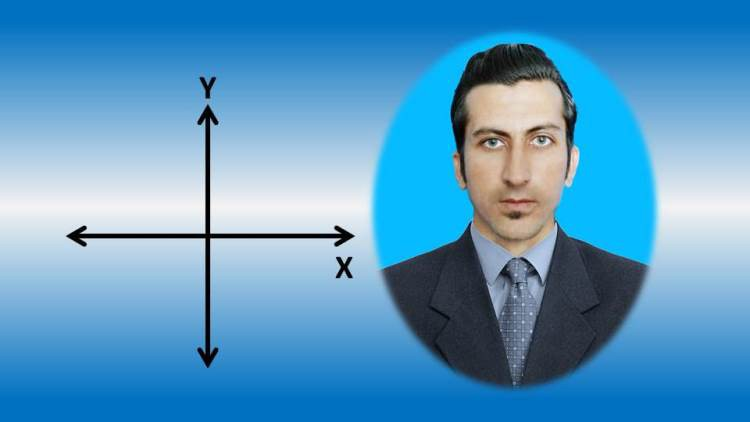# Fundamentals of Mathematics

Fundamental of Mathematics(particularly algebra) through animation,explanations,plenty of solved examples for exam
SETS: Introduction, Names, Types and Forms of sets. Solve numerical problems
SET OPERATIONS: Introduction, types, properties, laws of set operation.
DE MORGRAN'S LAWS: De Morgan's First Law and De Morgan's Second Law with relevant examples
VENN DIAGRAMS: Venn diagrams for subsets, disjoint, overlapping, complement and difference of sets. Then some solved numericals
BINARY OPERATIONS: Introduction, Properties, Addition Laws, Multiplication Laws with applications and some solved problems
NUMBER SYSTEM: Real numbers, Complex numbers, Derivations, Verification, conjugate, modulus, Properties of equality & Inequality, Addition Laws, Multiplication Laws and some solved problems
COORDINATE SYSTEM: Cartesian coordinate system; x-y plane, complex plane, Polar coordinate system; real plane, complex plane. Some solved numerical problems
GROUPS: Categories; Groupoid, Monoid, Semi-Group, Group, Abelian Group. Some solved problems

Learn the Fundamental of Mathematics through animation. This course includes videos explanation starting right from introduction and basics, then takes graphical and numerical phase with formulas, verification and proofs. At the end it carries plenty of solved numerical problems with the relevant examples. The lectures’ videos are appealing, attractive, fancy (with some nice graphic designing), fast and take less time to walk you through the whole lecture. It’s a prefect choice for students who feel boredom watching long lectures and wants things to finish them quickly with the maximum knowledge gain. So join me here and do it in a quick and easy way. This course covers the below list of topics:

• Sets

• Introduction

• Names

• Types

• Forms

• Numerical problems

• Set Operations

• Introduction

• Types

• Properties

• Laws of set operation

• De Morgan’s Laws

• De Morgan’s First Law

• De Morgan’s Second Law

• Relevant examples

• Venn Diagrams

• Venn diagrams for subset of sets

• Venn diagrams for disjoint of sets

• Venn diagrams for overlapping of sets

• Venn diagrams for complement of sets

• Venn diagrams for difference of sets.

• Some solved problems

• Binary Operations

• Introduction

• Properties

• Addition Laws with applications

• Multiplication Laws with applications

• Some solved problems

• Numerical System

• Real numbers

• Complex numbers

• Derivations & Verification

• Conjugate of complex numbers

• modulus of complex numbers

• Properties of equality & Inequality

• Addition Laws

• Multiplication Laws

• Solved Problems

• Coordinate System

• Cartesian coordinate system

• X-Y plane

• Cartesian coordinate system

• X-Y plane

• Complex plane

• Polar coordinate system

• Real plane & complex plane

• Solved numerical problems

• Groups

• Categories

• Groupoid

• Monoid

• Semi-Group

• Group

• Abelian Group

• Some solved problems

You can view and review the lecture materials indefinitely, like an on-demand channel.
Definitely! If you have an internet connection, courses on Udemy are available on any device at any time. If you don't have an internet connection, some instructors also let their students download course lectures. That's up to the instructor though, so make sure you get on their good side!

#### Be the first to add a review.

Please, login to leave a reviewCourse available for 2 days
30-Day Money-Back Guarantee

#### Includes

12 hours on-demand video
Full lifetime access
Access on mobile and TV
Certificate of Completion
+ +